Next: CONSERVATION PRINCIPLES AND MODE Up: Mathematical physics in stratified Previous: UP- AND DOWNGOING WAVES

The principle of reciprocity states that a source and receiver may (under some conditions) be interchanged and the same waveform will be observed. This principle is often used to advantage in calculations and may also be used to simplify data collection. It is somewhat amazing that this principle applies t other earth with its complicated inhomogeneities. Intuitively, the main reason for validity of the reciprocal principle is that energy propagates equally well along a given ray in either direction. Either way, it goes at the same speed with the same attenuation. This is true for all common types of waves.

Little more would need to be said if all waves were scalar phenomena with scalar sources and scalar receivers as, for example, acoustic pressure waves with explosive sources and pressure-sensitive receivers. The situation becomes more complicated when the sources or receivers are moving diaphragms, because then their orientations become important. The directional properties of the source and receiver are often referred to as radiation patterns. To apply the reciprocity principle it is necessary to regard the radiation patterns as attached to the medium, not as being attached to the source and receiver. Thus, when source and receiver are said to be interchanged, it is only a scalar magnitude which is interchanged; the radiation patterns stay fixed at the same place. These general ideas are made more precise in the following derivation. It will be seen that the notion of rays actually turns out to be irrelevant. Reciprocity also works in diffusion and potential problems.

Theoretical treatments are often somewhat hard to read. They often begin by specifying that the differential operator along with suitable boundary conditions should constitute a self-adjoint problem. This means that when you reexpress the differential equations in difference form you discover that the matrix of coefficients is symmetric. Let us take the example of acoustic waves in one dimension. Newton's equation says that mass densitytimes accelerations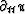equals the negative of the pressure gradient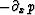plus the external force Fx. Utilizing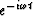time dependence we have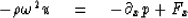which, defining F = - Fx, may be written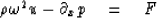(1)
The other important equation of acoustics says that the incompressibility K-1 multiplied by the pressure p plus the divergence of displacement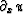equals the external (relative) volume injection V, that is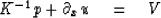(2)
We will now combine (9-4-1) and (9-4-2) in a finite difference form with, for convenience,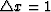.In practice, one might like to use many grid points to approximate the behavior of continuous functions, but for the sake of illustration we only need use a few grid points. Luckily, in this case reciprocity will be exactly true despite the small number of grid points. We have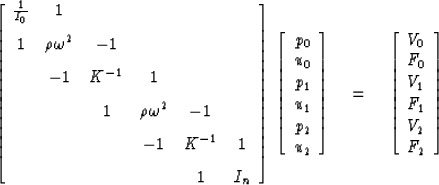(3)
The first and last rows of (9-4-3) requires some special comment. The quantities I0 and In are called impedances. If they vanish, we have zero pressure end conditions; if they are infinite, we have zero motion end conditions.

Now with all this fuss we have gone through to obtain the matrix (9-4-3), the only thing we want from it is to observe that the matrix is indeed a symmetric matrix (even ifand K-1 were functions of x). In the exercises it is shown that a symmetric matrix may also be attained in two dimensions. That the matrix is symmetric is partly a result of the physical nature of sound and partly a result of careful planning on the part of the author. To obtain the correct statement of reciprocity in other situations you may have to do some careful planning too. The essence of reciprocity is that since the matrix of (9-4-3) is symmetric then the inverse matrix will also be symmetric. Premultiplying (9-4-3) through by the inverse matrix we get the responses as a result of matrix multiplication on the external excitations.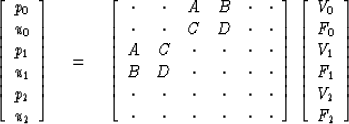(4)
The letters A, B, C, and D indicate the symmetry of the matrix of (9-4-4). Now if all external sources vanish except on one end where there is a unit strength volume source V0 = 1, then according to (9-4-4) the pressure in the middle p1 will equal A. If in a second experiment all the external sources vanish except the middle volume source V1 = 1, then according to (9-4-4) the pressure response p0 at the end will also equal A. This is the reciprocal principle. Note that with the letter D in (9-4-4) a like statement applies to the forces and the displacements. A mixed statement applies with the letters C and B.

In a realistic experiment it may not be possible to have a pure volume source or a pure external force. In other words, the external source may have some finite, nonzero impedance. Then the first experiment we would perform would be with the excitation at the middle, getting for the end response: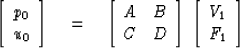(5)
Interchanging source and receiver locations, we have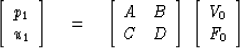(6)
The notable feature of (9-4-5) and (9-4-6) is that the matrices are transposes of one another. This feature would not be lost if we were to consider a more elaborate experiment where the vectors in (9-4-5) and (9-4-6) contained more elements. For example, a vector in (9-4-5) or (9-4-6) could contain elements of an array of physically separated volume sources or pressure sensors. In fact, if the reader is able to frame elastic, electromagnetic, diffusion, or potential problems as symmetric algebraic equations like (9-4-3), then the matrices like (9-4-5) and (9-4-6) will still be transposes of one another. The setting up of symmetric equations like (9-4-3) is often not difficult, although it may get somewhat complicated in multidimensional noncartesian geometry.

In such a more general case we may denote the right-hand vectors in (9-4-5) or (9-4-6) by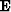to denote excitation and the left-hand vectors by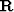to denote response. Using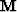for the matrix of (9-4-5) and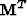for the transposed matrix, (9-4-5) and (9-4-6) would be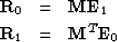(7) (8)

Now let us deduce a physical statement from (9-4-7) and (9-4-8). First take the inner product of (9-4-7) with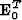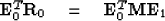The right-hand side, which is a scalar, may be transposed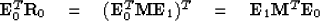substituting from (9-4-8) we have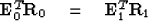(9)
Equation (9-4-9) is the basic statement of reciprocity; the inner product of the excitation vector and the response vector at place 0 equals their inner product at place 1. Notice that the inner products are between vectors which occur in different experiments.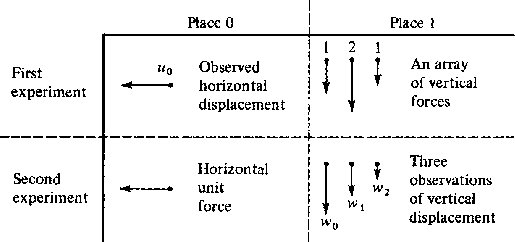9-2
Figure 2
A reciprocity example. Reciprocity says that u0 = w0 + 2w1 + w2.

An example of an elastic system with vector-directed displacement and force vectors is depicted in Figure 9-2. A laboratory example by J. E. White which combines electromagnetic, solid, liquid, and gaseous media is down in Figure 9-3. A geophone is a spring pendulum couped to an induction coil. The first geophone is mounted on a pipe which rests on the bottom of a glass desiccator. The second geophone is attached to the glass with a chunk of modeling clay, below the water level. The top pair of traces shows the (source) current into the first geophone and the (open circuit) voltage at the second; the bottom traces show the current in the second geophone and the voltage at the first.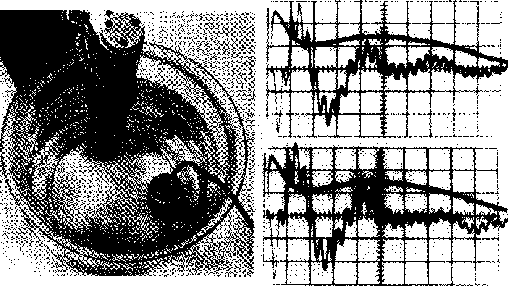9-3
Figure 3
An example of the reciprocal principle in a combined electromagnetic, solid, liquid, and gaseous system [J.E. White].

## EXERCISES:

1. Consider Poisson's equation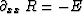on five grid points where the boundary conditions are that the end points are zero. A unit excitation at the third grid point gives the solution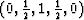.Find the solution with a unit excitation in the second grid point. Observe reciprocity if you do it right.
2. Write an equation like (9-4-3) for the heat-flow equation. How will the introduction of imaginary numbers change the statement of the reciprocal principle?
3. Write the three first-order partial differential equations of acoustics in two-dimensional cartesian geometry. Observe the gridding arrangement below.

Write a set of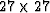equations for the vector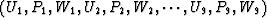.Make it come out symmetric and in an obviously orderly form.

4. In Sec 8-3, Exercises 5 and 6 taken together illustrate the reciprocity theorem which states, If source and receiver are interchanged, the same waveform will be observed.'' Solve the problem of a surface source with a receiver in the middle of the layers and solve the same problem with interchanged source and receiver to test the reciprocity theorems.Next: CONSERVATION PRINCIPLES AND MODE Up: Mathematical physics in stratified Previous: UP- AND DOWNGOING WAVES
Stanford Exploration Project
10/30/1997